# Standalone colorbars#

This tutorial shows how to build and customize standalone colorbars, i.e. without an attached plot.

A `colorbar` needs a "mappable" (`matplotlib.cm.ScalarMappable`) object (typically, an image) which indicates the colormap and the norm to be used. In order to create a colorbar without an attached image, one can instead use a `ScalarMappable` with no associated data.

```import matplotlib.pyplot as plt
import matplotlib as mpl
```

## Basic continuous colorbar#

Here, we create a basic continuous colorbar with ticks and labels.

The arguments to the `colorbar` call are the `ScalarMappable` (constructed using the norm and cmap arguments), the axes where the colorbar should be drawn, and the colorbar's orientation.

For more information see the `colorbar` API.

```fig, ax = plt.subplots(figsize=(6, 1), layout='constrained')

cmap = mpl.cm.cool
norm = mpl.colors.Normalize(vmin=5, vmax=10)

fig.colorbar(mpl.cm.ScalarMappable(norm=norm, cmap=cmap),
cax=ax, orientation='horizontal', label='Some Units')
```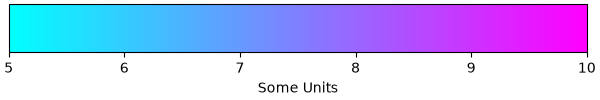## Colorbar attached next to a pre-existing axes#

All examples in this tutorial (except this one) show a standalone colorbar on its own figure, but it is possible to display the colorbar next to a pre-existing Axes ax by passing `ax=ax` to the colorbar() call (meaning "draw the colorbar next to ax") rather than `cax=ax` (meaning "draw the colorbar on ax").

```fig, ax = plt.subplots(layout='constrained')

fig.colorbar(mpl.cm.ScalarMappable(norm=mpl.colors.Normalize(0, 1), cmap='magma'),
ax=ax, orientation='vertical', label='a colorbar label')
```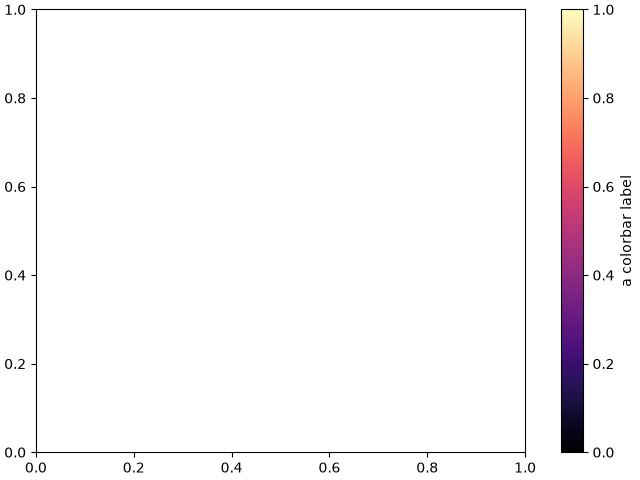## Discrete and extended colorbar with continuous colorscale#

The following example shows how to make a discrete colorbar based on a continuous cmap. We use `matplotlib.colors.BoundaryNorm` to describe the interval boundaries (which must be in increasing order), and further pass the extend argument to it to further display "over" and "under" colors (which are used for data outside of the norm range).

```fig, ax = plt.subplots(figsize=(6, 1), layout='constrained')

cmap = mpl.cm.viridis
bounds = [-1, 2, 5, 7, 12, 15]
norm = mpl.colors.BoundaryNorm(bounds, cmap.N, extend='both')

fig.colorbar(mpl.cm.ScalarMappable(norm=norm, cmap=cmap),
cax=ax, orientation='horizontal',
label="Discrete intervals with extend='both' keyword")
```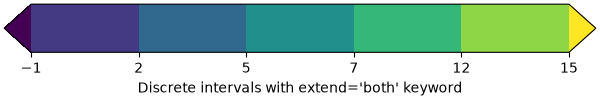## Colorbar with arbitrary colors#

The following example still uses a `BoundaryNorm` to describe discrete interval boundaries, but now uses a `matplotlib.colors.ListedColormap` to associate each interval with an arbitrary color (there must be as many intervals than there are colors). The "over" and "under" colors are set on the colormap using `Colormap.with_extremes`.

We also pass additional arguments to `colorbar`:

• To display the out-of-range values on the colorbar, we use the extend argument in the colorbar() call. (This is equivalent to passing the extend argument in the `BoundaryNorm` constructor as done in the previous example.)

• To make the length of each colorbar segment proportional to its corresponding interval, we use the spacing argument in the colorbar() call.

```fig, ax = plt.subplots(figsize=(6, 1), layout='constrained')

cmap = (mpl.colors.ListedColormap(['red', 'green', 'blue', 'cyan'])
.with_extremes(under='yellow', over='magenta'))
bounds = [1, 2, 4, 7, 8]
norm = mpl.colors.BoundaryNorm(bounds, cmap.N)

fig.colorbar(
mpl.cm.ScalarMappable(cmap=cmap, norm=norm),
cax=ax, orientation='horizontal',
extend='both',
spacing='proportional',
label='Discrete intervals, some other units',
)
```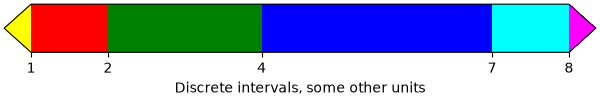## Colorbar with custom extension lengths#

We can customize the length colorbar extensions, on a colorbar with discrete intervals. To make the length of each extension the same as the length of the interior colors, use `extendfrac='auto'`.

```fig, ax = plt.subplots(figsize=(6, 1), layout='constrained')

cmap = (mpl.colors.ListedColormap(['royalblue', 'cyan', 'yellow', 'orange'])
.with_extremes(over='red', under='blue'))
bounds = [-1.0, -0.5, 0.0, 0.5, 1.0]
norm = mpl.colors.BoundaryNorm(bounds, cmap.N)

fig.colorbar(
mpl.cm.ScalarMappable(cmap=cmap, norm=norm),
cax=ax, orientation='horizontal',
extend='both', extendfrac='auto',
spacing='uniform',
label='Custom extension lengths, some other units',
)

plt.show()
```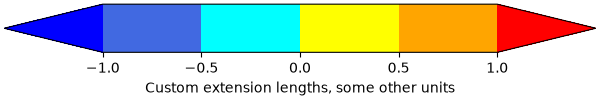Gallery generated by Sphinx-Gallery